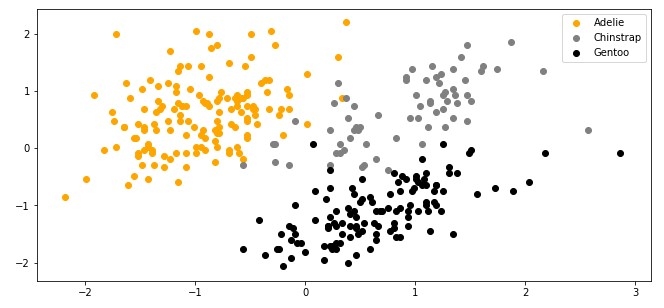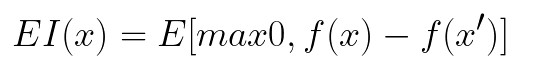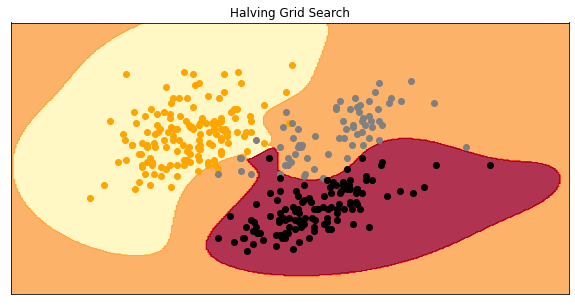Select Page

* indicates required

In a previous couple of articles, we were specifically focused on machine learning algorithms’ performance. We talked about how to quantify machine learning model performance and how to improve it with regularization. Apart from that, we covered the topic optimization techniques, both basic ones like Gradient Descent and advanced ones, like Adam.

It is pretty surreal how completely different sub-branches grew around the concept of model optimization. One of those sub-branches is hyperparameter optimization or hyperparameter tuning.

This bundle of e-books is specially crafted for beginners. Everything from Python basics to the deployment of Machine Learning algorithms to production in one place. Become a Machine Learning Superhero TODAY!

## 1. Hyperparameters in Machine Learning

Hyperparameters are an integral part of every machine learning and deep learning algorithm. Unlike standard machine learning parameters that are learned by the algorithm itself (like w and b in linear regression, or connection weights in a neural network), hyperparameters are set by the engineer before the training process.

They are an external factor that controls the behavior of the learning algorithm fully defined by the engineer. Do you need some examples? The learning rate is one of the most famous hyperparameters, C in SVM is also a hyperparameter, maximal depth of Decision Tree is a hyperparameter, etc. These can be set manually by the engineer.However, if we want to run multiple tests, this can be tiresome. That is where we use hyperparameter optimization. The main goal of these techniques is to find the hyperparameters of a given machine learning algorithm that deliver the best performance as measured on a validation set. In this tutorial, we explore several techniques that can give you the best hyperparameters.

## 2. Prerequisites & Data

### 2.1 Prerequisites and Libraries

For the purpose of this article, make sure that you have installed the following Python libraries:

• NumPy – Follow this guide if you need help with installation.
• SciKit Learn – Follow this guide if you need help with installation.
• SciPy – Follow this guide if you need help with installation.
• Sci-Kit Optimization – Follow this guide if you need help with installation.

Once installed make sure that you have imported all the necessary modules that are used in this tutorial.

``````import pandas as pd
import numpy as np
import matplotlib.pyplot as plt

from sklearn.preprocessing import StandardScaler
from sklearn.model_selection import train_test_split
from sklearn.metrics import f1_score

from sklearn.model_selection import GridSearchCV, RandomizedSearchCV

from sklearn.experimental import enable_halving_search_cv
from sklearn.model_selection import HalvingGridSearchCV, HalvingRandomSearchCV

from sklearn.svm import SVC
from sklearn.ensemble import RandomForestRegressor

from scipy import stats
from skopt import BayesSearchCV
from skopt.space import Real, Categorical``````

Apart from that, it would be good to be at least familiar with the basics of linear algebra, calculus and probability.

### 2.2 Preparing Data

Data that we use in this article is from PalmerPenguins Dataset. This dataset has been recently introduced as an alternative to the famous Iris dataset. It is created by Dr. Kristen Gorman and the Palmer Station, Antarctica LTER. You can obtain this dataset here, or via Kaggle.

This dataset is essentially composed of two datasets, each containing data of 344 penguins. Just like in Iris dataset there are 3 different species of penguins coming from 3 islands in the Palmer Archipelago. Also, these datasets contain culmen dimensions for each species. The culmen is the upper ridge of a bird’s bill. In the simplified penguin’s data, culmen length and depth are renamed as variables culmen_length_mm and culmen_depth_mm.Since this dataset is labeled, we will be able to verify the result of our experiments. However, this is often not the case and validation of clustering algorithm results is often a hard and complicated process.

``````data = pd.read_csv('./data/penguins_size.csv')

data = data.dropna()
data = data.drop(['sex', 'island', 'flipper_length_mm', 'body_mass_g'], axis=1)``````

Then we separate input data and scale it:

``````X = data.drop(['species'], axis=1)

ss = StandardScaler()
X = ss.fit_transform(X)

y = data['species']
spicies = {'Adelie': 0, 'Chinstrap': 1, 'Gentoo': 2}
y = [spicies[item] for item in y]
y = np.array(y) ``````

Finally, we split data into training and testing datasets:

``X_train, X_test, y_train, y_test = train_test_split(X, y, test_size=0.2, random_state=33)``

When we plot the data here is what it looks like:## 3. Grid Search Hyperparameter Tuning

Manual hyperparameter tuning is slow and tiresome. That is why we explore the first and simplest hyperparameters optimization technique – Grid Search. This technique is speeding up that process and it is one of the most used hyperparameter optimization techniques. In its essence, it automates the trial and error process. For this technique, we provide a list of all hyperparameter values and this algorithm builds a model for each possible combination, evaluates it, and selects values that provide the best results. It is a universal technique that can be applied to any model.In our example, we use SVM algorithm for classification. There are three hyperparameters that we take into consideration – C, gamma and kernel. To understand them in more detail, check out this article. For C we want to check the following values: 0.1, 1, 100, 1000; for gamma we use values: 0.0001, 0.001, 0.005, 0.1, 1, 3, 5, and for kernel we use values: ‘linear’ and ‘rbf’

### 3.1 Grid Search Implementation

Here is how that looks like in the code:

``````hyperparameters = {
'C': [0.1, 1, 100, 1000],
'gamma': [0.0001, 0.001, 0.005, 0.1, 1, 3, 5],
'kernel': ('linear', 'rbf')
}``````

We utilize Sci-Kit Learn and its SVC class which contains the implementation of SVM for classification. Apart from that, we use GridSearchCV class, which is used for grid search optimization. Combined that looks like this:

``````grid = GridSearchCV(
estimator=SVC(),
param_grid=hyperparameters,
cv=5,
scoring='f1_micro',
n_jobs=-1)``````

This class receives several parameters through the constructor:

• estimator – the instance machine learning algorithm itself. We pass the new instance of the SVC class there.
• param_grid – contains hyperparameter dictionary.
• cv – Determines the cross-validation splitting strategy.
• scoring – The validation metrics used to evaluate the predictions. We use F1 score.
• n_jobs – Represents the number of jobs to run in parallel. Value -1 means that is using all processors.The only thing left to do is run the training process, by utilizing the fit method:

``grid.fit(X_train, y_train)``

Once training is complete, we can check the best hyperparameters and the score of those parameters:

``````print(f'Best parameters: {grid.best_params_}')
print(f'Best score: {grid.best_score_}')``````
``````Best parameters: {'C': 1000, 'gamma': 0.1, 'kernel': 'rbf'}
Best score: 0.9626834381551361		``````

Also, we can print out all the results:

``print(f'All results: {grid.cv_results_}')``
``````Allresults: {'mean_fit_time': array([0.00780015, 0.00280147, 0.00120015, 0.00219998, 0.0240006 ,
0.00739942, 0.00059962, 0.00600033, 0.0009994 , 0.00279789,
0.00099969, 0.00340114, 0.00059986, 0.00299864, 0.000597  ,
0.00340023, 0.00119658, 0.00280094, 0.00060058, 0.00179944,
0.00099964, 0.00079966, 0.00099916, 0.00100031, 0.00079999,
0.002     , 0.00080023, 0.00220037, 0.00119958, 0.00160012,
0.02939963, 0.00099955, 0.00119963, 0.00139995, 0.00100069,
0.00100017, 0.00140052, 0.00119977, 0.00099974, 0.00180006,
0.00100312, 0.00199976, 0.00220003, 0.00320096, 0.00240035,
0.001999  , 0.00319982, 0.00199995, 0.00299931, 0.00199928,
...``````

Ok, let’s now build this model and check how well it performs on the test dataset:

``````model = SVC(C=500, gamma = 0.1, kernel = 'rbf')
model.fit(X_train, y_train)

preditions = model.predict(X_test)
print(f1_score(preditions, y_test, average='micro'))``````
``0.9701492537313433``

Cool, our model with the proposed hyperparameters got the accuracy ~97%. Here is what the model looks like when plotted:## 4. Random Search Hyperparameter Tuning

Grid search is super simple. However, it is also computing expensive. Especially in the area of deep learning, where training can take a lot of time. Also, it can happen that some of the hyperparameters are more important than others. That is why the idea of Random Search was born and introduced in this paper. In fact, this study shows that random search is more efficient than the grid search for hyperparameter optimization in terms of computing costs. This technique allows the more precise discovery of good values for the important hyperparameters too.Just like Grid Search, Random Search creates a grid of hyperparameter values and selects random combinations to train the model. It’s possible for this approach to miss the most optimal combinations, however, it surprisingly picks the best result more often than not and in a fraction of the time compared to Grid Search

### 4.1 Random Search Implementation

Let’s see how that works in the code. Again we utilize Sci-Kit Learn’s SVC class, but this time we use RandomSearchCV class for random search optimization.

``````hyperparameters = {
"C": stats.uniform(500, 1500),
"gamma": stats.uniform(0, 1),
'kernel': ('linear', 'rbf')
}

random = RandomizedSearchCV(
estimator = SVC(),
param_distributions = hyperparameters,
n_iter = 100,
cv = 3,
random_state=42,
n_jobs = -1)

random.fit(X_train, y_train)``````

Note that we used uniform distribution for C and gamma. Again, we can print out the results:

``````print(f'Best parameters: {random.best_params_}')
print(f'Best score: {random.best_score_}')``````
``````Best parameters: {'C': 510.5994578295761, 'gamma': 0.023062425041415757, 'kernel': 'linear'}
Best score: 0.9700374531835205``````

Note that we got close, but different results than when we used Grid Search. The value of the hyperparameter C was 500 with Grid Search, while with Random Search we got 510.59. From this alone, you can see the benefit of Random Search, since it is unlikely that we would put this value in the grid search list. Similarly for the gamma, we got 0.23 for Random Search against 0.1 for Grid Search. What is really surprising is that Random Search picked linear kernel and not RBF and that it got a higher F1 Score with it. To print all results we use the cv_results_ attribute:

``print(f'All results: {random.cv_results_}')``
``````Allresults: {'mean_fit_time': array([0.00200065, 0.00233404, 0.00100454, 0.00233777, 0.00100009,
0.00033339, 0.00099715, 0.00132942, 0.00099921, 0.00066725,
0.00266568, 0.00233348, 0.00233301, 0.0006667 , 0.00233285,
0.00100001, 0.00099993, 0.00033331, 0.00166742, 0.00233364,
0.00199914, 0.00433286, 0.00399915, 0.00200049, 0.01033338,
0.00100342, 0.0029997 , 0.00166655, 0.00166726, 0.00133403,
0.00233293, 0.00133729, 0.00100009, 0.00066662, 0.00066646,

....``````

Let’s do the same thing as we did fro Grid Search: create the model with proposed hyperparameters, check the score on the test dataset and plot out the model.

``````model = SVC(C=510.5994578295761, gamma = 0.023062425041415757, kernel = 'linear')
model.fit(X_train, y_train)

preditions = model.predict(X_test)
print(f1_score(preditions, y_test, average='micro'))``````
``0.9701492537313433``

Wow, the F1 score on test dataset is exactly the same as when we used Grid Search. Check out the model:## 5. Bayesian Hyperparameter Optimization

Really cool fact about the previous two algorithms is that all he experiments with various hyperparameter values can be run in parallel. This can save us a lot of time. However, this is also their biggest lack. Meaning, since every experiment is run in isolation, we can not use the information from past experiments in the current one. There is a whole field that is dedicated to the problem of sequenced optimization – sequential model-based optimization (SMBO). Algorithms that are explored in this field use previous experiments and observations of the loss function. Based on them they try to determine the next optimal point. One of such algorithms is Bayesian Optimisation.Just like other algorithms from the SMBO group use previously evaluated points (in this case those are hyperparameter values, but we can generalize) to compute the posterior expectation of what the loss function looks like. This algorithm uses two important math concepts – The Gaussian process and acquisition function. Since Gaussian distribution is done over random variables, Gaussian process is its generalization over functions. Just like Gaussian distribution has mean value and covariance, the Gaussian process is described by mean function and covariance function.

The acquisition function is the function using which we evaluate the current loss value. One way to observe it is as a loss function for loss function. It is a function of the posterior distribution over loss function, that describes the utility for all values of the hyperparameters. The most popular acquisition function is expected improvement:where f is loss function, x’ is the current optimal set of hyperparameters. When we put it all together Byesian optimization is done in 3 steps:

• Using previously evaluated points for loss function, the posterior expectation is calculated using Gaussian Process.
• New set of points that maximizes expected improvent is chosen
• Loss function of new selected points is calculated### 5.1 Bayesian Optimization Implementation

The easies way to bring this to code is by using Sci-Kit optimization library, often called skopt. Following the process that we used on pravious examples, we can do the following:

``````hyperparameters = {
"C": Real(1e-6, 1e+6, prior='log-uniform'),
"gamma": Real(1e-6, 1e+1, prior='log-uniform'),
"kernel": Categorical(['linear', 'rbf']),
}

bayesian = BayesSearchCV(
estimator = SVC(),
search_spaces = hyperparameters,
n_iter = 100,
cv = 5,
random_state=42,
n_jobs = -1)

bayesian.fit(X_train, y_train)``````

Again, we defined dictionary for set of hyperparameters. Note that we used Real and Categorical classes from Sci-Kit Optimisation library. Then we utilize BayesSearchCV class in the same way we used GridSearchCV or RandomSearchCV. After the training is done, we can print out the best results:

``````print(f'Best parameters: {bayesian.best_params_}')
print(f'Best score: {bayesian.best_score_}')``````
``````Best parameters:
OrderedDict([('C', 3932.2516133086), ('gamma', 0.0011646737978730447), ('kernel', 'rbf')])
Best score: 0.9625468164794008``````

It is interesting, istn’t it? We got quite different results using this optimization. Loss is a bit higher than when we used Random Search. We can even print out all results:

``print(f'All results: {bayesian.cv_results_}')``
``````All results: defaultdict(<class 'list'>, {'split0_test_score': [0.9629629629629629,
0.9444444444444444, 0.9444444444444444, 0.9444444444444444,  0.9444444444444444,
0.9444444444444444, 0.9444444444444444, 0.9444444444444444, 0.46296296296296297,
0.9444444444444444, 0.8703703703703703, 0.9444444444444444, 0.9444444444444444,
0.9444444444444444, 0.9444444444444444, 0.9444444444444444, 0.9444444444444444,
.....``````

How the model with these hyperparameters performs on the test dataset? Let’s find out:

``````model = SVC(C=3932.2516133086, gamma = 0.0011646737978730447, kernel = 'rbf')
model.fit(X_train, y_train)

preditions = model.predict(X_test)
print(f1_score(preditions, y_test, average='micro'))``````
``0.9850746268656716``

This is super interesting. We got better score on test dataset even though we got worse results on validation dataset. Here is the model:Just for fun, let’s put all these models side by side:## 6. Halving Grid Search & Halving Random Search

A couple of months ago Sci-Kit Learn introduced two new classes HalvingGridSearchCV and HalvingRandomSearchCV. They claimed that with these two classes they claimed that “they can be much faster at finding a good parameter combination”. These classes used search over specified parameter values with successive halving. This technique starts evaluating all the candidates with a small number of resources and iteratively selects the best candidates, using more and more resources.From the point of Halving Grid Search, this means that in the first iteration all candidates will be trained on the small amount of training data. The next iteration would include only candidates that performed the best in the previous iteration. These models would get more resources, ie. more training data and they would be evaluated. This process would continue, and Halving Grid Search would keep only the best candidates from the previous iterations until there is only one left.

This whole process is controlled by two arguments — min_samples and factor. The first argument – min_samples represents the amount of data that the process will start with. With each iteration, this dataset will grow by the value defined by the factor. The process is similar to HalvingRandomSearchCV.

### 6.1 Halving Grid Search & Halving Random Search Implementation

The code is similar like in previous examples, we just use different classes. Let’s start with HalvingGridSearch:

``````hyperparameters = {
'C': [0.1, 1, 100, 500, 1000],
'gamma': [0.0001, 0.001, 0.01, 0.005, 0.1, 1, 3, 5],
'kernel': ('linear', 'rbf')
}

grid = HalvingGridSearchCV(
estimator=SVC(),
param_grid=hyperparameters,
cv=5,
scoring='f1_micro',
n_jobs=-1)

grid.fit(X_train, y_train)``````

The interesting thing is that this code run in just 0.7 seconds. In comparison, the same code with GridSearchCV class lasts 3.6 seconds. That is much faster. The results are a bit different though:

``````print(f'Best parameters: {grid.best_params_}')
print(f'Best score: {grid.best_score_}')``````
``````Best parameters: {'C': 500, 'gamma': 0.005, 'kernel': 'rbf'}
Best score: 0.9529411764705882``````

We got similar results, but not the same. If we create a model with these values, we will get the following accuracy and graph:

``````model = SVC(C=500, gamma = 0.005, kernel = 'rbf')
model.fit(X_train, y_train)

preditions = model.predict(X_test)
print(f1_score(preditions, y_test, average='micro'))``````
``0.9850746268656716``We do completely the same thing with Halving Random Search. It is interesting that with this approach we got the weirdest results. We may say that model created this way is overfitting hard:

``````hyperparameters = {
"C": stats.uniform(500, 1500),
"gamma": stats.uniform(0, 1),
'kernel': ('linear', 'rbf')
}

random = HalvingRandomSearchCV(
estimator = SVC(),
param_distributions = hyperparameters,
cv = 3,
random_state=42,
n_jobs = -1)

random.fit(X_train, y_train)

print(f'Best parameters: {random.best_params_}')
print(f'Best score: {random.best_score_}')``````
``````Best parameters: {'C': 530.8767414437036, 'gamma': 0.9699098521619943, 'kernel': 'rbf'}
Best score: 0.9506172839506174``````## 7. Alternatives

In general, previously described methods are the most popular and the most frequently used. However, there are several alternatives that you can consider if previous ones are not working out for you. One of them is Gradient-Based optimization of hyperparameter values. This technique calculates the gradient with respect to hyperparameters and then optimizes them using the gradient descent algorithm. The problem with this approach is that for gradient descent to work well we need function that is convex and smooth, which is often not the case when we talk about hyperparameters. The other approach is the use of Evolutionary algorithms for optimization.

## Conclusion

In this article, we covered several well known hyperparameter optimization and tuning algorithms. We learned how we can use Grid search, random search and bayesian optimization to get best values for our hyperparameters. We also saw how we can utilize Sci-Kit Learn classes and methods to do so in code.

This bundle of e-books is specially crafted for beginners. Everything from Python basics to the deployment of Machine Learning algorithms to production in one place. Become a Machine Learning Superhero TODAY!#### Nikola M. Zivkovic

Nikola M. Zivkovic is the author of books: Ultimate Guide to Machine Learning and Deep Learning for Programmers. He loves knowledge sharing, and he is an experienced speaker. You can find him speaking at meetups, conferences, and as a guest lecturer at the University of Novi Sad.

Ultimate Guide to Machine Learning with Python

Everything from Python basics to the deployment of Machine Learning algorithms to production in one place.

Become a Machine Learning Superhero TODAY!